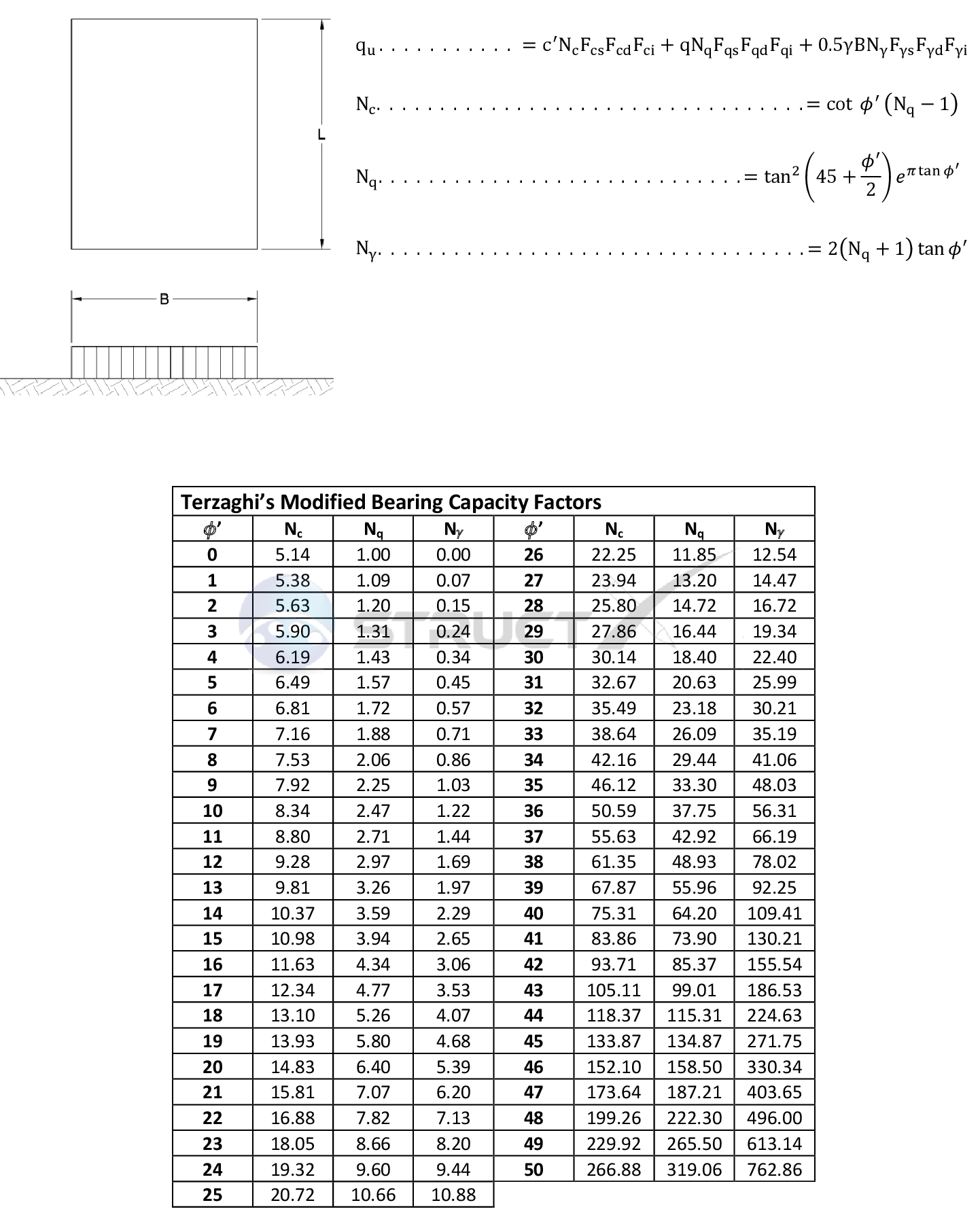# The General Bearing Capacity Equation - Rectangular FoundationsMore CasesNote: When calculating the shape, depth and inclination factors the effective width B' should not be used.
For foundations with one-way eccentricities.
For foundations with two-way eccentricities.

# Notation and Units

## Metric and Imperial Units

The above formulas may be used with both imperial and metric units. As with all calculations care must be taken to keep consistent units throughout with examples of units which should be adopted listed below:

# Notation

• B = width of foundation, ft or m
• L = length of foundation (where L > B), ft or m
• c' = effective cohesion of soil, lb/ft2 or kN/m2
• Df = depth of ground foundation measured from ground surface, ft or m
• Fcs, Fqs, Fγs = shape factors
• Fcd, Fqd, Fγd = depth factors
• Fci, Fqi, Fγi = load inclination factors
• Nc, Nq, Nγ = bearing capacity factors
• q = effective unit weight of soil (refer modification for a water table), lb/ft3 or kN/m3
• qu = ultimate bearing capacity, lb/ft2 or kN/m2 (kPa)
• γ = unit weight of soil, lb/ft3 or kN/m3
• ϕ' = effective angle of internal friction, degrees